Each side of a square is increasing at a rate of 4 cm/s. At what rate (in cm2/s) is the area of the square increasing when the area of the s

Question

Each side of a square is increasing at a rate of 4 cm/s. At what rate (in cm2/s) is the area of the square increasing when the area of the square is 25 cm2

in progress 0
6 months 2021-07-28T14:36:20+00:00 1 Answers 3 views 0

The area of the square is increasing at a rate of 40 square centimeters per second.

Step-by-step explanation:

The area of the square (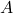), in square centimeters, is represented by the following function: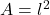(1)

Whereis the side length, in centimeters.

Then, we derive (1) in time to calculate the rate of change of the area of the square (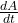), in square centimeters per second: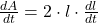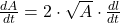(2)

Where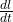is the rate of change of the side length, in centimeters per second.

If we know that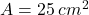and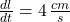, then the rate of change of the area of the square is: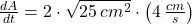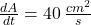The area of the square is increasing at a rate of 40 square centimeters per second.